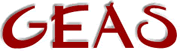[ < ] [ > ] [ << ] [ Up ] [ >> ] [Top] [Contents] [Index] [ ? ]

#### 1.2.13.1 How to declare and use arrays

Arrays really aren't arrays in the proper sense of the word. They can better be seen as lists with fixed order. The difference might seem slight, but it makes sense to the computer-science buffs :)

Arrays are type-specific. This means that an array of a certain type only can contain variables of that single type. Another restrictions is that all arrays are one-dimensional. You can't have an array of arrays. However, the `mixed` type takes care of these limitations. A mixed variable can act as an array containing any data type, even other arrays. As a rule you should try to use properly typed arrays to minimize the probabilities of programming mistakes however.

You declare an array like this:

 ``` *; e.g. int *my_arr, *your_arr; float *another_arr; object *ob_arr; ```

The initial values of these declared arrays is '0', not an empty array. I repeat: they are initialized to 0 and not to an empty array. Keep this in mind!

You can allocate and initialize an array like this:

 ``` = ({ elem1, elem2, elem3, ..., elemN }); e.g. my_arr = ({ 1, 383, 5, 391, -4, 6 }); ```

You access members of the array using brackets on the variable name. (Assume val here is declared to be an integer).

 ``` = []; e.g. val = my_arr; ```

LPC, like C, starts counting from 0, making the index to the fourth value = 3.

To set the value of an existing position to a new value, simply set it using the `=` operator.

 ``` my_arr = 22; // => ({ 1, 383, 5, 22, -4, 6 }) my_arr = 391; // => ({ 1, 383, 5, 391, -4, 6 }) ```

If you want to make a subset of an array you can specify a range of indices within the brackets.

 ``` = [..]; e.g. your_arr = my_arr[1..3]; ```

... will result in `your_arr` becoming the new array ```({ 383, 5, 391 });``` If you give a new value to an old array, the previous array is lost.

 ```e.g. my_arr = ({ }); ```

... will result in `my_arr` holding an empty array. The old array is de-allocated and the memory previously used is reclaimed by the gamedriver.

If you index outside an array, an error occurs and execution of the object is aborted. However, range indexing outside the array does not result in an error, the range is then only constrained to fall within the array.

If you want to create an empty array, initialized to 0 (no matter the type of the array, all positions will be set to 0 anyway) of a given length, you use the efun `allocate()`.

 ``` = allocate(); e.g. your_arr = allocate(3); // => your_arr = ({ 0, 0, 0 }); ```

Concatenating (adding) arrays to each other is most easily done with the `+` operator. Simply add them as you would numbers. The `+=` operator works fine as well.

 ```my_arr = ({ 9, 3 }) + ({ 5, 10, 3 }); // => ({ 9, 3, 5, 10, 3 }) ```

Removing elements from an array is easiest done with the `-/-=` operator, however, be aware that it is a general operator that will remove all items found that match the item you want to remove.

 ```my_arr -= ({ 3, 10 }); // => ({ 9, 5 }) ```

If you want to remove a single item in the middle somewhere that might have been repeated, you have to use the range operator of course.

 ```my_arr = ({ 9, 3, 5, 10, 3 }); my_arr = my_arr[0..0] + my_arr[2..4]; // => ({ 9, 5, 10, 3 }) ```

NB! Beware this difference!!!! One is a list, the other an integer!

 ``` my_arr[0..0] // = ({ 9 }) my_arr // = 9 ```

 [ < ] [ > ] [ << ] [ Up ] [ >> ] [Top] [Contents] [Index] [ ? ]

This document was generated by Ronny Wikh on July, 8 2003 using texi2html